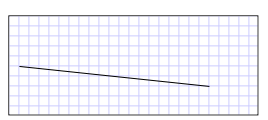# How to: Create a LineSegment in a PathGeometry

This example shows how to create a line segment. To create a line segment, use the PathGeometry, PathFigure, and LineSegment classes.

## Example

The following examples draw a LineSegment from (10, 50) to (200, 70). The following illustration shows the resulting LineSegment; a grid background was added to show the coordinate system.A LineSegment drawn from (10,50) to (200,70)

In Extensible Application Markup Language (XAML), you may use attribute syntax to describe a path.

``````<Path Stroke="Black" StrokeThickness="1"
Data="M 10,50 L 200,70" />
``````

(Note that this attribute syntax actually creates a StreamGeometry, a lighter-weight version of a PathGeometry. For more information, see the Path Markup Syntax page.)

In XAML, you may also draw a line segment by using object element syntax. The following is equivalent to the previous XAML example.

``````<Path Stroke="Black" StrokeThickness="1">
<Path.Data>
<PathGeometry>
<PathFigure StartPoint="10,50">
<LineSegment Point="200,70" />
</PathFigure>
</PathGeometry>
</Path.Data>
</Path>
``````
``````PathFigure myPathFigure = new PathFigure();
myPathFigure.StartPoint = new Point(10, 50);

LineSegment myLineSegment = new LineSegment();
myLineSegment.Point = new Point(200, 70);

PathSegmentCollection myPathSegmentCollection = new PathSegmentCollection();

myPathFigure.Segments = myPathSegmentCollection;

PathFigureCollection myPathFigureCollection = new PathFigureCollection();

PathGeometry myPathGeometry = new PathGeometry();
myPathGeometry.Figures = myPathFigureCollection;

Path myPath = new Path();
myPath.Stroke = Brushes.Black;
myPath.StrokeThickness = 1;
myPath.Data = myPathGeometry;
``````
``````Dim myPathFigure As New PathFigure()
myPathFigure.StartPoint = New Point(10, 50)

Dim myLineSegment As New LineSegment()
myLineSegment.Point = New Point(200, 70)

Dim myPathSegmentCollection As New PathSegmentCollection()

myPathFigure.Segments = myPathSegmentCollection

Dim myPathFigureCollection As New PathFigureCollection()